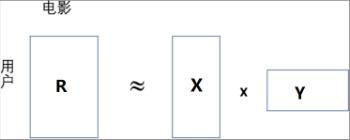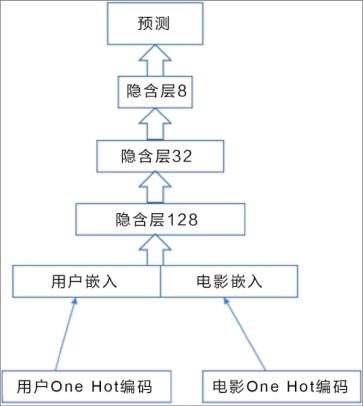# 推荐系统是如何建立模型、知道用户爱好的？

## 管理员账号

2017-09-25### 1.矩阵分解模型### 2.深度神经网络模型1 k = 128
2 model1 = Sequential()
3 model1.add(Embedding(n_users + 1, k, input_length = 1))
5 model2 = Sequential()
6 model2.add(Embedding(n_movies + 1, k, input_length = 1))

1 model = Sequential()
2 model.add(Merge([model1, model2], mode = ‘concat’))

model.compile(loss = ‘mse’, optimizer = “adam”)

1 users = ratings[‘user_id’].values
2 movies = ratings[‘movie_id’].values
5 推荐系统

label = ratings[‘rating’].values

1 X_train = [users, movies]
2 y_train = label

model.fit(X_train, y_train, batch_size = 100, epochs = 50)

1 i,j = 10,99
2 pred = model.predict([np.array([users[i]]), np.array([movies[j]])])

1 sum = 0
2 for i in range(ratings.shape):
3 sum += (ratings[‘rating’][i] - model.predict([np.array([ratings[‘user_id’
][i]]), np.array([ratings[‘movie_id’][i]])])) ** 2
4 mse = math.sqrt(sum/ratings.shape)
5 print(mse)

• ## 推荐系统是如何建立模型、知道用户爱好的？

小编说：你是否有过这样的经历？当你在亚马逊商城浏览一些书籍，或者购买过一些书籍后，你的偏好就会被系统学到，系统会基于一些假设为你推荐相关书目。为什么系统会知道，在这背后又藏着哪些秘密呢？本文选自《Keras快速上手：基于Python的...

1048 0 0 0
• ## #小编推书#唯一一本以应用为导向的介绍机器学习和深度学习的专业书籍

《Keras快速上手：基于Python的深度学习实战》从如何准备深度学习的环境开始，手把手地教读者如何采集数据，如何运用一些最常用，也是目前被认为最有效的一些深度学习算法来解决实际问题。覆盖的领域包括推荐系统、图像识别、自然语言情感分析...

714 0 0 0
• ## Keras 文字生成系统

同是深度学习“槛内人”，我怎么不知道这样高大上的文字生成对话系统 文字信息是存在最广泛的信息形式之一，而深度学习的序列模型（Sequential Model）在对文字生成建模（Generative Model）方面具备独特的优势。...

1622 0 0 0